# Conversion Relations of Trigonometric Ratios

Conversion Relations of Trigonometric Ratios

## Introduction:

Coterminal angles are angles in standard position (angles with the initial side on the positive  $$x$$-axis) that have a common terminal side.  For example, $$30^\circ$$, $$- 330^\circ$$ and $$390^\circ$$ are all coterminal.

Note: To find a positive and a negative angle coterminal with a given angle, you can add and subtract $$360^\circ$$ if the angle is measured in degrees or $$2\pi$$ if the angle is measured in radians.

Let's discuss some more conversion relations of trigonometric ratios.

## Conversion Relations:

What will be the value of $$\sin \left( { - \theta } \right)$$ in terms of $$\sin \theta$$? Consider the following figure: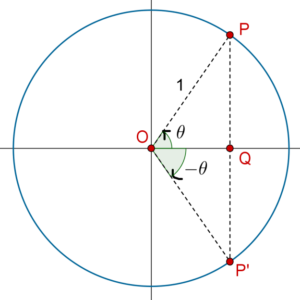We have:

\begin{align}&\sin \theta = \frac{{PQ}}{{OP}} = \frac{{PQ}}{1} = PQ\\&\sin \left( { - \theta } \right) = \frac{{P'Q}}{{OP'}} = \frac{{PQ}}{1} = PQ\end{align}

Thus,

$\boxed {\sin \left( { - \theta } \right) = \sin \theta }$

This also means that

$\boxed{\text{cosec}\,\left( { - \theta } \right) = \text{cosec}\,\theta}$

What about $$cos\left( { - \theta } \right)$$? From the figure above, we have

\begin{align}&\cos \theta = \frac{{OQ}}{{OP}} = \frac{{OQ}}{1} = OQ\\&cos\left( { - \theta } \right) = \frac{{OQ}}{{OP'}} = \frac{{OQ}}{1} = OQ\end{align}

Thus,

\boxed{\begin{align}&\cos\left( { - \theta } \right) = \cos \theta \\&\sec \left( { - \theta } \right) = \sec \theta \end{align}}

Finally, from the same figure, we have:

\begin{align}\tan \theta &= \frac{{PQ}}{{OQ}} \\\tan \left( { - \theta } \right) &= \frac{{P'Q}}{{OQ}} = \frac{{PQ}}{{OQ}}\end{align}

Thus,

\boxed{\begin{align}&\tan \left( { - \theta } \right) = - \tan \theta \\&\cot \left( { - \theta } \right) = - \cot \theta \end{align}}Challenge 1: Find the values of (a)  $$\sin \left( { - \frac{\pi }{6}} \right)$$  (b)  $$\cos \left( { - \frac{\pi }{3}} \right)$$  (c)  $$\tan \left( { - \frac{\pi }{4}} \right)$$.

Tip: Use conversion relation of $$\sin \left( { - \theta } \right)$$, $$\cos \left( { - \theta } \right)$$, and $$\tan \left( { - \theta } \right)$$.

We have already seen the following complementary angle relations (we used the degree scale earlier instead of the radian scale):

\begin{align}&\sin \left( {\frac{\pi }{2} - \theta } \right) = \cos \theta ,\,\,\,\cos \left( {\frac{\pi }{2} - \theta } \right) = \sin \theta \\&\tan \left( {\frac{\pi }{2} - \theta } \right) = \cot \theta ,\,\,\,\cot \left( {\frac{\pi }{2} - \theta } \right) = \tan \theta \\&\sec \left( {\frac{\pi }{2} - \theta } \right) = {\mathop{\rm \text{cosec}}\nolimits}\, \theta ,\,\,\,\text{cosec}\,\left( {\frac{\pi }{2} - \theta } \right) = \sec \theta \end{align}

We justified these relations for $$\theta$$ between 0 and $$\frac{\pi }{2}$$ radians. However, they hold true for $$\theta$$ of any arbitrary magnitude. Can you see how?

These relations above are just few of the many possible such relations which trigonometric ratios satisfy. For example, consider the following relation:

$\sin \left( {\frac{\pi }{2} + \theta } \right) = \cos \theta$

Let us prove this relation for acute $$\theta$$. Consider the following figure: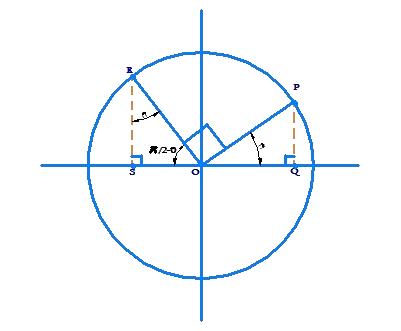Note: $$\Delta OQP$$ and $$\Delta RSO$$ are congruent, which means that $$OQ = RS$$ and $$OS = PQ$$. We have:

\begin{align}&\sin \left( {\frac{\pi }{2} + \theta } \right) = \frac{{RS}}{{RO}} = RS = OQ\\&\cos \theta = \frac{{OQ}}{{OP}} = OQ\\&\Rightarrow \boxed{\sin \left( {\frac{\pi }{2} + \theta } \right) = \cos \theta }\end{align}

Using the same figure, we can prove that,

\begin{align}&\cos \left( {\frac{\pi }{2} + \theta } \right) = - \sin \theta \\&\tan \left( {\frac{\pi }{2} + \theta } \right) = - \cot \theta \end{align}

Let us prove the first of these relations. We have:

\begin{align}&\cos \left( {\frac{\pi }{2} + \theta } \right) = \frac{{OS}}{{OR}} = \frac{{ - PQ}}{1} = - PQ\\& - \sin \theta = - \frac{{PQ}}{{OP}} = - PQ\\& \Rightarrow \,\,\,\cos \left( {\frac{\pi }{2} + \theta } \right) = - \sin \theta \end{align}

Next, consider the following set of relations:

\begin{align}&\sin \left( {\pi + \theta } \right) = - \sin \theta \\&\cos \left( {\pi + \theta } \right) = - \cos \theta \\&\tan \left( {\pi + \theta } \right) = \tan \theta \end{align}

Can you prove these? Consider the following figure: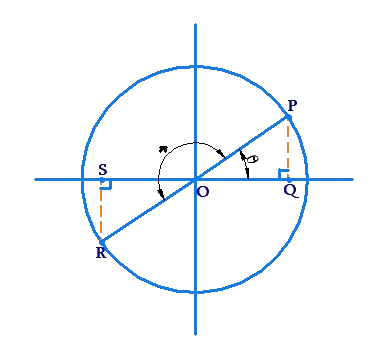The point corresponding to the angle $$\pi + \theta$$ is diametrically opposite to the point corresponding to the angle $$\theta$$. We have:

\begin{align}&\sin \left( {\pi + \theta } \right) = \frac{{RS}}{{OR}} = \frac{{ - PQ}}{1} = - PQ = - \sin \theta \\&\cos \left( {\pi + \theta } \right) = \frac{{OS}}{{OR}} = \frac{{ - OQ}}{1} = - OQ = - \cos \theta \\&\tan \left( {\pi + \theta } \right) = \frac{{RS}}{{OS}} = \frac{{ - PQ}}{{ - OQ}} = \frac{{PQ}}{{OQ}} = \tan \theta \end{align}

## Solved Examples:

Example 1: Prove geometrically that:

\begin{align}&\sin \left( {\pi - \theta } \right) = \sin \theta \\&\cos \left( {\pi - \theta } \right) = - \cos \theta \\&\tan \left( {\pi - \theta } \right) = - \tan \theta \end{align}

Solution:Consider the following figure: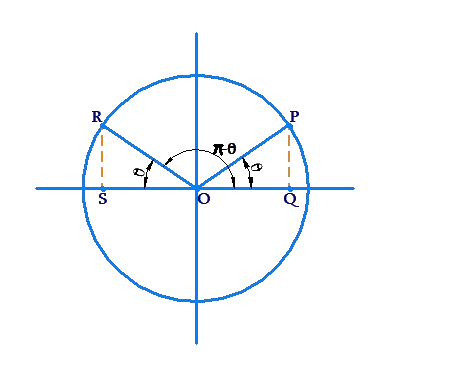We have:

\begin{align}& \sin \left( {\pi - \theta } \right) = \frac{{RS}}{{OR}} = \frac{{PQ}}{1} = PQ = \sin \theta \\ &\cos \left( {\pi - \theta } \right) = \frac{{OS}}{{OR}} = \frac{{ - OQ}}{1} = - OQ = - \cos \theta \\ &\tan \left( {\pi - \theta } \right) = \frac{{RS}}{{OS}} = \frac{{PQ}}{{ - OQ}} = - \frac{{PQ}}{{OQ}} = - \tan \theta \end{align}

Example 2: Prove geometrically that:

\begin{align}&\sin \left( {2\pi - \theta } \right) = - \sin \theta \\&\cos \left( {2\pi - \theta } \right) = \cos \theta \\&\tan \left( {2\pi - \theta } \right) = - \tan \theta \end{align}

Solution: Consider the following figure: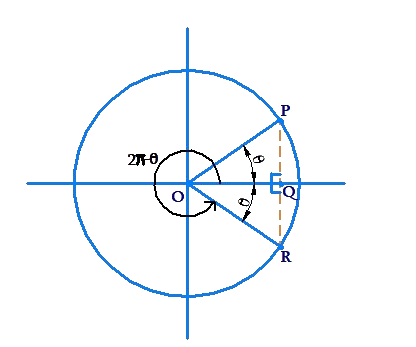We have:

\begin{align}&\sin \left( {2\pi - \theta } \right) = \frac{{RQ}}{{OR}} = \frac{{ - PQ}}{1} = - PQ = - \sin \theta \\&\cos \left( {2\pi - \theta } \right) = \frac{{OQ}}{{OR}} = \frac{{OQ}}{1} = OQ = \cos \theta \\&\tan \left( {2\pi - \theta } \right) = \frac{{RQ}}{{OQ}} = \frac{{ - PQ}}{{OQ}} = - \frac{{PQ}}{{OQ}} = - \tan \theta \end{align}Challenge 2: Prove geometrically that:

\begin{align}&\sin \left( {2\pi + \theta } \right) = \sin \theta \\&\cos \left( {2\pi + \theta } \right) = \cos \theta \\&\tan \left( {2\pi + \theta } \right) = \tan \theta \end{align}

Tip: Show visually that the angle $$2\pi + \theta$$ geometrically corresponds to the same configuration as the angle $$\theta$$, and hence the trigonometric ratios of these angles are the same.

Example 3: Express the value of $$\sin \left( {\frac{{11\pi }}{2} + \theta } \right)$$ in terms of a trigonometric ratio of  $$\theta$$.

Solution: We have:

\begin{align}&\sin \left( {\frac{{11\pi }}{2} + \theta } \right) = \sin \left( {6\pi - \frac{\pi }{2} + \theta } \right)\\&\qquad\qquad\qquad\; = \sin \left( { - \frac{\pi }{2} + \theta } \right)\\&\qquad\qquad\qquad\; = - \sin \left( {\frac{\pi }{2} - \theta } \right) = \cos \theta \end{align}

Example 4: Express the value of $$\tan \left( {19\pi - \theta } \right)$$ in terms of a trigonometric ratio of  $$\theta$$.

Solution: We proved earlier that $$\tan \left( {\pi + \theta } \right)$$ is the same as $$\tan \theta$$. Thus, adding any multiple of $$\pi$$ to the original argument will not change the original $$\tan$$ value. This means that:

$\tan \left( {19\pi - \theta } \right) = \tan \left( { - \theta } \right) = - \tan \theta$Challenge 3: Express the value of $$\cos \left( {\frac{{9\pi }}{2} + \theta } \right)$$ in terms of a trigonometric ratio of  $$\theta$$.

Tip: Use a similar approach as in example-3.

Trigonometry
Trigonometry
Grade 9 | Questions Set 1
Trigonometry
Grade 10 | Questions Set 1
Trigonometry
More Important Topics
Numbers
Algebra
Geometry
Measurement
Money
Data
Trigonometry
Calculus
More Important Topics
Numbers
Algebra
Geometry
Measurement
Money
Data
Trigonometry
Calculus
Learn from the best math teachers and top your exams

• Live one on one classroom and doubt clearing
• Practice worksheets in and after class for conceptual clarity
• Personalized curriculum to keep up with school